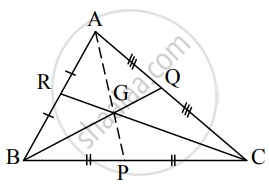# Prove that medians of a triangle are concurrent - Mathematics and Statistics

Sum

Prove that medians of a triangle are concurrent

#### Solution

Consider ∆ABC.

Let P, Q, R be the midpoints of the sides BC, CA, AB respectively.

Let the medians BQ and CR intersect at G.

To prove that the third median AP also passes through G.

Let bar"a", bar"b", bar"c", bar"p", bar"q", bar"r", bar"g" be the position vectors of the points A, B, C, P, Q, R, G respectively.

Since P, Q, R are the mid-points of the sides BC, CA, AB respectively∴ By midpoint formula, we get

bar"p" = (bar"b" + bar"c")/2   .......(i)

bar"q" = (bar"c" + bar"a")/2   .......(ii)

bar"r" = (bar"a" + bar"b")/2   .......(iii)

From (i), (ii) and (iii), we get

2bar"p" =bar"b" + bar"c" ⇒ 2bar"p" + bar"a" = bar"a" + bar"b" + bar"c"

2bar"q" = bar"c" + bar"a" ⇒ 2bar"q" + bar"b" = bar"a" + bar"b" + bar"c"

2bar"r" = bar"a" + bar"b" ⇒ 2bar"r" + bar"c" = bar"a" + bar"b" + bar"c"

∴ (2"p" + bar"a")/3 = (2bar"q" + bar"b")/3 = (2bar"r" + bar"c")/3 = (bar"a" + bar"b" + bar"c")/3

∴ (2"p" + bar"a")/(2 + 1) = (2bar"q" + bar"b")/(2 + 1) = (2bar"r" + bar"c")/(2 +1) = (bar"a" + bar"b" + bar"c")/3

= bar"g"    .......(say)

This shows that the point G whose position vector is bar"g" lies on the three medians AP, BQ, CR dividing them internally in the ratio 2:1.

Hence, the three medians are concurrent.

Concept: Section Formula
Is there an error in this question or solution?